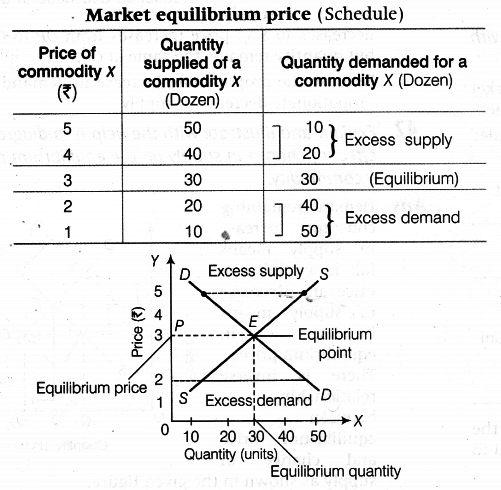# Explain how market price of a good is determined using diagram

Explain how market price of a good is determined using diagram.
or
How is price determined under perfect competition? Explain briefly.
or
How is equilibrium price of a commodity determined? Explain with the help of demand and supply schedule.

Market price or equilibrium price is determined by the forces of market demand and market supply. Considering market demand schedule on one hand and market supply schedule on the other, we identify equilibrium price as the one where market demand is equal to market supply or where market demand curve and market supply curve intersect each other.In the above schedule and diagram, demand and supply become equal only at the price of Rs. 3.00, so it will be our equilibrium price. Also, it is clear that equilibrium price is determined at the point, where demand and supply curves intersect each other at 30 units of commodity ‘X’.